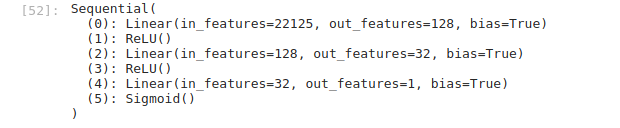# Optimization of performance

Hello,

I am relatively new to deep learning with PyTorch. I tried to build a multi-layer feed-forward neural network that takes a tf-idf vector of a news title and outputs 0 or 1 whether the title is fake or not. The dataset used for the task was the onion dataset. Unfortunately, the part of preprocessing cannot change. So any optimization can be done on the model’s architecture. My code is this:

``````import sys
import time
import nltk
import numpy
import pandas
import torch.nn
import statistics
import torch.utils
import sklearn.metrics
import sklearn.model_selection
import sklearn.feature_extraction.text

# preprocessing
tokenized_vector = dict()
for i in input_dataframe.index:
tokenized_vector[i] = nltk.word_tokenize(input_dataframe.loc[i])
stemmer = nltk.PorterStemmer()
stopwords = set(nltk.corpus.stopwords.words('english'))
for tokens in tokenized_vector:
for counter, token in enumerate(tokenized_vector[tokens]):
if stemmer.stem(tokenized_vector[tokens][counter]) not in stopwords:
tokenized_vector[tokens][counter] = stemmer.stem(tokenized_vector[tokens][counter])
else:
tokenized_vector[tokens].remove(tokenized_vector[tokens][counter])
vectorizer = sklearn.feature_extraction.text.TfidfVectorizer()
preprocessed_tokenized_vector = list()
for i in tokenized_vector:
preprocessed_tokenized_vector.append(' '.join(tokenized_vector[i]))
X = vectorizer.fit_transform(preprocessed_tokenized_vector)
tf_idf_df = pandas.DataFrame(X.todense(), columns=vectorizer.get_feature_names(), dtype=numpy.float16)
preprocessed_data = pandas.concat([tf_idf_df, input_dataframe.iloc[:, 1:]], axis=1, sort=False)
print('Total size of dataframe: ', round(sys.getsizeof(preprocessed_data) / 2**20, 2), 'MB')
del X
del tf_idf_df
del preprocessed_tokenized_vector
del tokenized_vector
del input_dataframe
cols = pandas.DataFrame(preprocessed_data.columns[:-1].tolist(), columns=['tokens'])

# initialize dataset
X = preprocessed_data.iloc[:, :-1]
Y = pandas.concat([preprocessed_data.iloc[:, -1], abs(preprocessed_data.iloc[:, -1] - 1)], axis=1).astype(numpy.int8)
del preprocessed_data
Y.columns = ['valid', 'fake']
x_fit, x_test, y_fit, y_test = \
sklearn.model_selection.train_test_split(X, Y,
test_size=0.25, random_state=42)
x_train, x_val, y_train, y_val = \
sklearn.model_selection.train_test_split(x_fit,
y_fit,
test_size=0.10,
random_state=42)
del x_fit
del y_fit

# prepare data for pytorch
x_train = torch.from_numpy(x_train.to_numpy()).float()
y_train = torch.from_numpy(y_train.to_numpy()).float()
train_dataset = torch.utils.data.TensorDataset(x_train, y_train)
del x_train
del y_train
x_val = torch.from_numpy(x_val.to_numpy()).float()
y_val = torch.from_numpy(y_val.to_numpy()).float()
val_dataset = torch.utils.data.TensorDataset(x_val, y_val)
del x_val
del y_val
x_test = torch.from_numpy(x_test.to_numpy()).float()
y_test = torch.from_numpy(y_test.to_numpy()).float()
test_dataset = torch.utils.data.TensorDataset(x_test, y_test)
del x_test
del y_test
del train_dataset
del val_dataset
del test_dataset
X = torch.from_numpy(X.to_numpy()).float()
Y = torch.from_numpy(Y.to_numpy()).float()

# model architecture - This part must be optimized
model = torch.nn.Sequential(
torch.nn.Linear(X.shape, 1024),
torch.nn.ReLU(),
torch.nn.Linear(1024, 512),
torch.nn.ReLU(),
torch.nn.Linear(512, 2),
torch.nn.Softmax(dim=1)
)
criterion = torch.nn.BCELoss()
learning_rate = 1e-5
def get_device():
device = None
if torch.cuda.is_available():
device = torch.device('cuda')
else:
device = torch.device('cpu')
return device
device = get_device()
model.to(device)
early_stopping = False
prevent = 5
consecutive = False
message = ' '
epoch = 0
epochs = 50
prev_mean_valid_loss = numpy.Inf
start = 0
train_loss = []
valid_loss = []
history = []

#fit model
print('Time: ', start, ' (in seconds)')
while not early_stopping and epoch < epochs:
if epoch == 0:
start = time.time()

# prep model for training
model.train()
# forward pass
y_hat = model(x_train.to(device))
# calculate the loss
loss = criterion(y_hat, y_train.to(device))
# clear the gradients of all optimized variables
# backward pass
loss.backward()
# perform a single optimization step (parameter update)
optimizer.step()
# update running training loss
train_loss.append(loss.item())
# shut down autograd to begin evaluation
# prep model for evaluation
model.eval()
# forward pass
y_hat = model(x_val.to(device))
# calculate the loss
loss = criterion(y_hat, y_val.to(device))
# update running validation loss
valid_loss.append(loss.item())
# early stopping conditional
if prev_mean_valid_loss <= statistics.mean(valid_loss):
if consecutive is True:
prevent -= 1
consecutive = True
if prevent < 0:
early_stopping = True
message = '\tPrevious average Validation error was lower than\
current Validation error'
else:
consecutive = False

# print results after 2 epochs
if epoch % 2 == 1:
end = time.time()
print('Epoch: ', epoch+1, '\t Time: +', end-start, '\t Training\
loss: ', statistics.mean(train_loss), '\t Validation loss: ',
statistics.mean(valid_loss))
start = time.time()

# update epoch's validation loss variable
prev_mean_valid_loss = statistics.mean(valid_loss)

# early stopping message
if early_stopping is True:
print('\t\tStopping at epoch: ', epoch + 1, message)
epoch = epochs - 1
epoch += 1

# test model
test_loss = []
# initialize timer
start = time.time()
# test model
model.eval()
yhat = model(x_test.to(device))
loss = criterion(yhat, y_test.to(device))
test_loss.append(loss.item())
# end time checkpoint
end = time.time()
# print test results
print('\tTime: {:.10} \tTest Loss: {:.15f}'.format(end-start,
statistics.mean(test_loss)))

``````

The problem is that the model’s architecture seems to be too “heavy”. Is there any possibility that the architecture of the model could be optimized in memory and computation complexity?

If you want to reduce the model capacity, you could lower the number of hidden neurons in the model.

Also note that `nn.BCELoss` is expecting a single neuron with a sigmoid applied on it for a binary classification use case.
So you would have to change the last linear layer to `nn.Linear(512, 1)` and use `nn.Sigmoid. Or remove the softmax and sigmoid, return the raw logits, and use `nn.BCEWithLogitsLoss`.

If you want to use two neurons for the binary classification, you could keep the last linear layer, remove the softmax, and use `nn.CrossEntropyLoss`.

Thank you very much ! My code is now executable. I changed the output layer of my model and there is now only one neuron that uses Sigmoid activation. I also reduced the number of hidden neurons. The new model architecture can be examined below:There is one small bit that is still ambiguous. The validation loss increases in every epoch, while the training loss decreases. Are there any more problems?